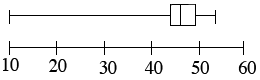### Home > CC2MN > Chapter 11 > Lesson 11.1.3 > Problem11-44

11-44.

Hollyhocks are tall, slender, flowering plants that grow in many areas of the U.S. Here are the heights (in inches) of hollyhocks that are growing in a park: $10$, $39$, $43$, $45$, $46$, $47$, $48$, $48$, $49$, $50$, and $52$.

1. Find the median.

Since the data is already listed in ascending order, find the middle number.

$47$

2. Find the quartiles.

Find the medians of the upper half and the lower half of the ordered set of data.

3. Make a box plot of the data.Use the eTool below to make a box plot.
Click the link at right for the full version of the eTool: 11-44 HW eTool (Desmos)